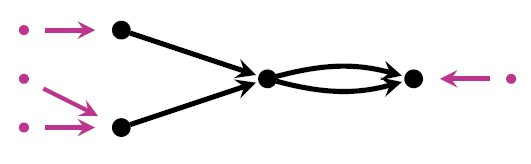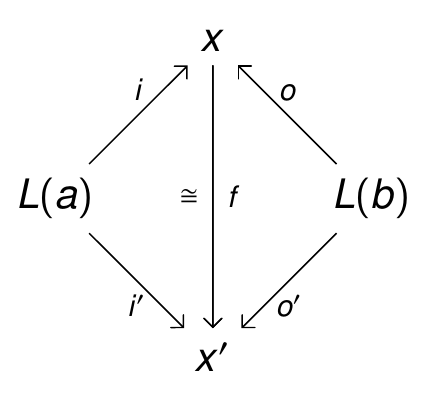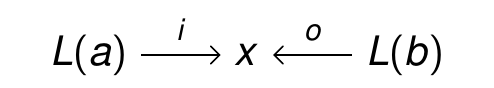## Structured Cospans

My grad student Kenny Courser gave a talk at the 4th Symposium on Compositional Structures. He spoke about his work with Christina Vasilakopolou and me. We’ve come up with a theory that can handle a broad class of open systems, from electrical circuits to chemical reaction networks to Markov processes and Petri nets. The idea is to treat open systems as morphisms in a category of a particular kind: a ‘structured cospan category’.

Here is our first paper on this subject:

• John Baez and Kenny Courser, Structured cospans, Theory and Applications of Categories 35 (2020), 1771–1822.

Here is his talk:

• Kenny Courser, Structured cospans.

In July 11th I’m going to talk about structured cospans at the big annual category theory conference, CT2019:

• John Baez, Structured cospans.

I borrowed more than just the title from Kenny’s talk… but since I’m an old guy, they’re giving me time to say more stuff. For full details, try Kenny’s thesis:

• Kenny Courser, Open Systems: A Double Categorical Perspective.

We’ve been working on this project for a couple of years, so there’s a lot to say… but right now let me just tell you what a ‘structured cospan’ is.

Suppose you have any functor$L \colon \mathsf{A} \to \mathsf{X}.$ Then a structured cospan is a diagram like this:For example if$L \colon \mathsf{A} \to \mathsf{X}$ is the functor from sets to graphs sending each set to the graph with that set of vertices and no edges, a structured cospan looks like this:It’s a graph with two sets getting mapped into its set of vertices. I call this an open graph. Or if$L \colon \mathsf{A} \to \mathsf{X}$ is the functor from sets to Petri nets sending each set to the Petri having that set of places and nothing else, a structured cospan looks like this:• John Baez, Open Petri nets, Azimuth, 15 August 2018.

It illustrates many ideas from the general theory of structured cospans: for example, what we do with them.

You may have heard of a similar idea: ‘decorated cospans’, invented by Brendan Fong. You may wonder what’s the difference!

Kenny’s talk explains the difference pretty well. Basically, decorated cospans that look isomorphic may not be technically isomorphic. For example, if we have an open graph like this:and its set of edges is$\{a,b,c,d\},$ this is not isomorphic to the identical-looking open graph whose set of edges is$\{b,c,d,e\}.$ That’s right: the names of the edges matter!

This is an annoying glitch in the formalism. As Kenny’s talk explains, structured cospans don’t suffer from this problem.

My talk at CT2019 explains another way to fix this problem: using a new improved concept of decorated cospan! This new improved concept gives results that match those coming from structured cospan in many cases. Proving this uses some nice theorems proved by Kenny Courser, Christina Vasilakopoulou and also Daniel Cicala.

But I think structured cospans are simpler than decorated cospans. They get the job done more easily in most cases, though they don’t handle everything that decorated cospans do.

I’ll be saying more about structured cospans as time goes on. The basic theorem, in case you’re curious but don’t want to look at my talk, is this:

Theorem. Let$\mathsf{A}$ be a category with finite coproducts,$\mathsf{X}$ a category with finite colimits, and$L \colon \mathsf{A} \to \mathsf{X}$ a functor preserving finite coproducts. Then there is a symmetric monoidal category${}_L \mathsf{Csp}(\mathsf{X})$ where:

• an object is an object of$\mathsf{A}$
• a morphism is an isomorphism class of structured cospans:Here two structured cospans are isomorphic if there is a commutative diagram of this form:If you don’t want to work with isomorphism classes of structured cospans, you can use a symmetric monoidal bicategory where the 1-morphisms are actual structured cospans. But following ideas of Mike Shulman, it’s easier to work with a symmetric monoidal double category. So:

Theorem. Let$\mathsf{A}$ be a category with finite coproducts,$\mathsf{X}$ a category with finite colimits, and$L \colon \mathsf{A} \to \mathsf{X}$ a functor preserving finite coproducts. Then there is a symmetric monoidal double category${_L \mathbb{C}\mathbf{sp}(\mathsf{X})}$ where:

• an object is an object of$\mathsf{A}$
• a vertical 1-morphism is a morphism of$\mathsf{A}$
• a horizontal 1-cell is a structured cospan• a 2-morphism is a commutative diagram### 4 Responses to Structured Cospans

1.Allen Knutson says:

Why is that category “Rex”?

•John Baez says:

“Rex” means “right exact”, which is the way abelian category theorists say “preserving finite colimits”. So cognoscenti in a rush say “rex functor” for “functor preserving finite colimits”, and use “Rex” for the category of categories with finite colimits and rex functors between these. Here I’m using it for the 2-category where we include natural transformations.

The analogous term “lex” is more commonly used, since “finite limits theories” are important in categorical algebra.

What’s the opposite of Tyrannosaurus rex?

2. Here’s a paper on categories where the morphisms are open physical systems, and composing them describes gluing these systems together:

• John C. Baez, David Weisbart and Adam Yassine, Open systems in classical mechanics.

The basic idea is by now familiar to fans of this blog—but there are some big twists! I like treating open systems as cospans with extra structure. But in this case it makes more sense to use spans, since the phase space of a classical system maps to the phase space of any subsystem. We’ll compose these spans using pullbacks.

3. My student Kenny Courser‘s thesis has hit the arXiv:

• Kenny Courser, Open Systems: A Double Categorical Perspective, Ph.D. thesis, U. C. Riverside, 2020.

This site uses Akismet to reduce spam. Learn how your comment data is processed.